Short Questions and Answers on Probability, Random Signals and Random Process Questions and Answers

Q.1. What are random signals? What is significance of random signals in probability theory?

Ans. There is one other class of signals, the behaviour of which cannot be predicted. Such type of signals are called random signals.

These signals are called random signals because the precise value of these signals cannot be predicted in advance before they actually occur.

The examples of random signals are the noise interference in communication systems. This means that the noise interference during transmission is totally unpredictable.

In the same way, the noise generated by the receiver itself is random. Even some other signals which are not noise signals are also random signals. These signals cannot be modelled mathematically. Actually the electromagnetic interference is the major source of random noise.

Q.2. What do you mean by Experiment in probability theory?

Ans. An experiment is defined as the process which is conducted to get some results. If the same experiment is performed repeatedly under the same conditions, similar results are expected. An experiment is sometimes called trial. As an example, throw of a coin is an experiment or trial. This trial results in two outcomes namely Head and Tail.

Q.3. What is sample space?

Ans. A set of all possible outcomes of an experiment or trial is called the sample space of that experiment.

It is generally denoted by ‘S’. The total number of outcomes in a sample space is denoted by n(s).

As an example, the tossing of a coin has two outcomes.

Hence, we may write its sample space as:

S = {H, T}

where, H -> Head and T -> Tail

Q.4. What is an probability theory?

Ans. The expected subset of the sample space or happening is called an event.

As an example, let us consider an experiment of throwing a cubic die. In this case, the sample space S will be as

S = {1, 2, 3, 4, 5, 6}

Now, if we want the number ‘3’ to be an outcome or an even number, i.e., {2, 4, 6}, then this subset is called an event.

This is denoted by letter ‘E’. Hence event E is a subset of the sample space ‘S’.

If event E has only one outcome, then it is called an elementary event.

On the other hand, if event E does not contain any out come, then it is called a null event.

If E = S, then an event contains all the outcomes. Such as event is called a certain event.

It always occurs, no matter what so ever is the outcome.

Q.5. What do you mean by probability? Explain.

Ans. Probability may be defined as the study of random experiments. In any random experiment, there is always an uncertainty that a particular event will occur or not. As a measure of probability of occurrence of an event, a number between 0 to 1 is assigned.

If it is sure that an event will occur, then we can say that its probability is 100% or 1.

If it is not sure that an event will occur, then we can say that its probability is 0% or 0.

If it is not sure whether the event will occur or not, then its probability is between 0 and 1.

Q.6. Write properties of probability? Explain.

Ans.

Property 1: The probability of a certain event is unity i.e.,

P (A) = 1

Property 2: The probability of any event is always less than or equal to 1 and non-negative. Mathematically,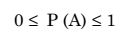Property 3: If A and B are two mutually exclusive events, then

P(A + B) = P(A) + P(B)

Property 4: If A is any event, then the probability of not happening of A is

P (Ā) = 1 – P (A)

where Ā represents the complement of event A.

Property 5: If A and B are any two events (not mutually exclusive events), then

P (A + B) = P (A) + P (B) – P (AB)

where P (AB) is called the probability of events A and B both occurring simultaneously. Such an event is called joint event of A and B, and the probability P (AB) is called the joint probability. Now, if events A and B are mutually exclusive, then the joint probability, P(AB) = 0.

Q.7. What is conditional probability?

Ans. The concept of conditional probability is used in conditional occurrences of the events.

Let us consider an experiment which involves two events A and B.

Now the probability of event B, given that event A has occurred, is represented by P (B/A).

Similarly, P (A/B) represents probability of event A given that event B has already occurred.

Therefore, P (B/A) and P (A/B) are called conditional probabilities.

Q.8. Explain random variable?

Ans. A function which can take on any value from the sample space and its range is some set of real numbers is called a random variable of the experiment.

Random variables are denoted by upper case letters such as X, Y etc., and the values taken by them are denoted by lower case letters with subscripts such as x1, x2, y1, y2 etc.

Random variables may be classified as under:

1. Discrete random variables

2. Continuous random variables.

Q.9. What is a continuous random variable?

Ans. A random variable that takes on an infinite number of values is called a continuous random variable.

Actually, there are several physical system (experiments) that generate continuous outputs or outcomes.

Such systems generate infinite number of outputs or outcomes within the finite period.

Continuous random variables may be used to define the outputs of such systems.

As an example, the noise voltage generated by an electronic amplifier has a continuous amplitude. This means that sample space S of the noise voltage amplitude is continuous. Therefore, in this case, the random variable X has a continuous range of values.

Q.10. What do you mean by probability density function (PDF)?

Ans. The derivative of cumulative distributive function (CDF) with respect to some dummy variable is known as Probability Density Function (PDF).

Probability density function (PDF) is generally denoted by fX(x).

Mathematically, PDF may be expressed as :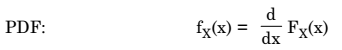where x is a dummy variable.

Probability density function (PDF) is the more convenient representation for continuous random variable.

Q.11. What do you mean by conditional probability density function?

Ans. Out of the two random variables, one variable may take a fixed value. In this case, the PDF is called conditional. As an example, out of the two continuous random variables X and Y, let X = x.

Then we may find the conditional, PDF of Y given that X = x as,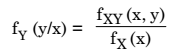where fX(x) is the marginal density of random variable X.

Similarly, we may find the conditional PDF of X given that Y = y.Then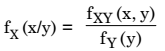Q.12. Explain uniform distribution?

Ans.  The Probability Density Function (PDF) of an uniform distribution is expressed as :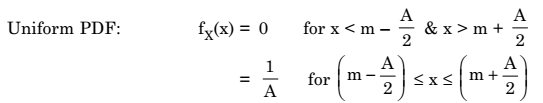Figure. 1 shows the PDF of an uniformly distributed random variable.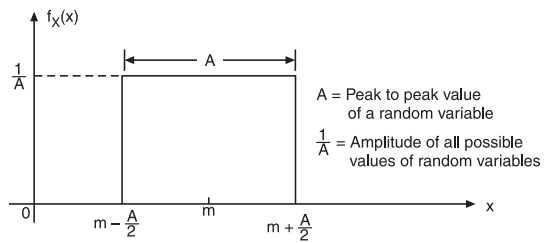Fig.1 : PDF of an uniformly distributed random variable

In above figure, the peak to peak value is A and amplitude is uniform i.e., A.

From figure.1, it is also clear that the random variable and its PDF are continuous. Therefore uniform distribution is utilized for continuous random variable. Also, the value of PDF, fX(x)(x) is same for all possible values of a random variable. Thus, this distribution is known as Uniform Distribution.

Q.13. What is mean or average?

Ans. The mean or average of any random variable is expressed by the summation of the values of random variables X weighted by their probabilities.

Mean value of a random variable is denoted by mx.

Mean value is also known as expected value of random variable X.

mx = E (X)

where E[] represents expection operator.

Therefore both mx and E (X) have the same meaning.

Also, in general term, mean or average is given by :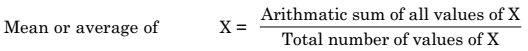Q.14. What is importance of Gaussian distribution?

Ans. The Gaussian distribution is used for continuous random variables. We know that the random motion of the thermally agitated electrons produces thermal noise. This thermal noise has Gaussian distribution. The random errors in the experimental measurements create the measured values to have Gaussian distribution about the true value. The Gaussian distribution is very important in the analysis of communication and statistical systems.

Q.15. What do you mean by random process? Explain.

Ans. Let there be a random experiment E having outcome λ from the sample space S. Thus every time an experiment is conducted, the outcome λ will be one of the sample point in sample space. If this outcome l is associated with time, then a function of λ and time t is formed i.e., X(λ, t). Then the function X(l, t) is known as random process.

Hence when any random experiment E is given a time dimension, then each outcome appears at some certain time and the random experiment will be converted to Random Process. A random process is the function of two variables λ and t.

Q.16. What is Ergodic process? Explain.

Ans. A random process is known as ergodic process if the time-averages are equal to ensemble averages. Hence for a ergodic process, we haveFor any ergodic process, all ensemble averages will be equal to corresponding time averages for any particular sample function. The time averages are not a function of time. When time and ensemble averages are the same, it implies that ensemble averages also are not a function of time. Thus an ergodic process is always stationary but converse in not true.

Ergodicity of the process may be defined in terms of some statistical averages like mean and autocorrelation.

The random process is ergodic in the mean if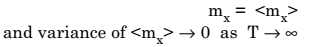Similarly, the random process is ergodic in the autocorrelation if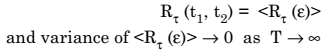Hence, the Ergodicity of any random process may be determined by evaluating statistical averages of single sample function. This means that a single sample function represents entire random process.